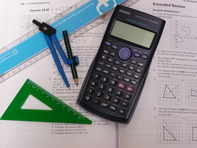# Maths 13-14

£165.00Pack includes: Student file, Textbook, CD for mental maths and 10 tutor marked assignments including complete SATs-type test.

Covers to level 7:

Numbers and Arithmetic, Approximations, Powers, Multiples, Factors and primes, Fractions and Percentages, Ratio, Proportion and Rates of Change, Algebraic Expressions, Equations and Inequalities, Formulas, Sequences, Graphs and Equations, Angles and Shapes, Constructions, Perimeter, Area and Volume, Transformations, Geometric Relationships (including Pythagoras and Trigonometry), Probability and Statistics.

:  at  £165.00  each